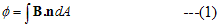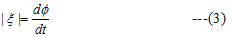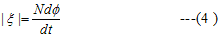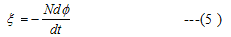# ElectroMagnetic induction

## (3) Magnetic Flux

• The flux of magnetic field through a surface is defined in a similar manner as we defined flux in the electric field
• if dA be an area element on any arbitrary surface and n be the unit vector perpendicular to the area element then magnetic flux is defined as• If the surface under considerations is a plane of area A and magnetic field is constant in both the magnitude and direction over the surface and θ is the angle between magnetic field B and unit normal to the surface then magnetic flux is given by
Φ=BAcosθ                                  --(2)
• Unit of flux is Wb(weber)
1Wb=1T.m2

## (4) Faradays law of electromagnetic induction

• From experiments on EM induction, faraday came to conclude then an emf was being induced in the coil when the magnetic flux linked to it was being changed either by
(i) Moving magnet close or away from the coil(experiment 1)
(ii) Chnaging amouny of current in the primary coil or motion of either of the coils relative to each other (experiment 2)
• From these observations faraday enunciated an important law. The magitude of the induced emf produced in the coil is given bywhere Φ is the magnetic flux as given by equation (1) .ξ is expressed in volts
• If the circuit is a tightly wound coil of N turns then total flux linked by N turns coil is NΦ.So induced EMF is whole coil isAbove equation only give the magnitude of the induced EMF and it does not give its direction.
• we know that unit of magnetic flux is 1 weber=1 T.m2
• If magnetic flux linking a single turn changes at a rate of 1weber per second then Induced emf=Magnetic flux/time=1 weber/sec Now since weber=Nm/Amp
Hence 1 weber/sec= 1 N.m/A.s =1 Joule/coulumb
• Hence the unit of emf is volt.

## (5) Direction of Induced EMF: Lenz's Law

• The direction of induced current and emf is given by lenz's law
• Due to change in magnetic flux through a closed loop an induced current is established in the loop
• Lenz's law states that
'The induced current due to the induced emf always flow in such a direction as to oppose the change causing it'
• Now we can combine faraday's law as given in equation (4) to find the direction of emf
• Thus we can say that "The emf induced in a coil is equal to the negative rate of the change of the magnetic flux linked with it "## Explaination of lenz's law

>
• To explain this law again consider faradays experiment 1 in which north pole of the magnet moves towards a closed coil
• This movement of north pole of magnetic induces current in the coil in such a direction so that end of the coil ,facing and approaching north pole becomes a magnetic north pole
• The repulsion between two poles opposes the motion of the magnet towards the coil
• Thus work has to be done to push the magnet against the coil
• It is this mechanical work which causes the current to flow in the coil against its resistance R and supply the energy for the heat loss
• The mechanical workdone is converted to electrical energy which produces the heat energy
• If the direction of the induced current were such as not to oppsose the motion ,then we would be obtaining electrical energy continously without doing any work ,which is impossible
• So ,every things seems to be all right if we accept lenz's law otherwise the principle of conservation of the energy would be voilated
• Direction of the induced current can be found using Fleming right hand rule.
" If we stretch thumb ,index and middle finger perpendicular to one another then index fingers points in direction of the magnetic field ,middle finger in direction of induced current and thum points in direction of the motion of the conducter"

Note to our visitors :-

Thanks for visiting our website.